Structural Bioinformatics Library Template C++ / Python API for developping structural bioinformatics applications.
User Manual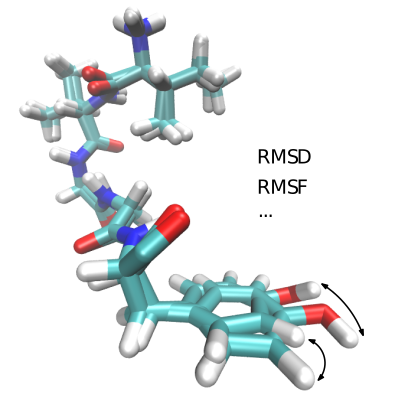# Molecular_distances

Authors: F. Cazals and T.Dreyfus

# Introduction

Goal. Molecular distances aim to compare different conformations of the same molecule or complex.

A conformation is represented either in Cartesian coordinates (using the Point_d format) or in internal coordinates. Computing distances between conformations occurs in essentially two situations:

• Conformations of a given molecule (or complex) yielded by a simulation method.
• Conformation of the same molecule (or complex) yielded by different experimental methods.

In the former case, it is clear that the same atoms are available. In the latter, the hydrogen atoms are typically not reported in a coherent way.

Modes. For distances based on Cartesian coordinates, this account for the following four modes used in distance calculations:

• Calpha atoms: distances are computed on Calpha.
• Backbone atoms: only Calpha, C and N atoms.
• Heavy atoms: distances are computed on all atoms but hydrogen atoms.
• All atoms: distances are computed on all atoms, including hydrogen atoms.
When comparing protein structures, it is safe to distinguish conformations versus polypeptide chains:
• Two conformations are characterized by the same triples { (chain id, res id, atom name) } for all the atoms involved in the comparison.
• For two sets of polypeptide chains from say two crystal structures obtained independently, the chain ids may not correspond to one another. For this reason, selected applications below (sbl-lrmsd-for-pdb-pair.exe, sbl-binet-cauchy-for-pad-pairs.exe) provide the option –skip-chain-ids. This option is typically used to compare two chains with different labels in two PDB files. This option should be used with care, though, since characterizing atoms solely based on (res id, atom name) can create duplicates if several chains are compared. (Nb: for such cases, a solution would consists of providing explicitly the mapping between chains.)

Two related packages are the following ones:
• Molecular_distances_flexible provides distances calculations with two refinements, as it handles (i) the so-called combined RMSD, and (ii) provides tools to process proteins whose sequences may differ – an alignment step is involved.
• Point_cloud_rigid_registration_3 provides the elementary geometric operations used in the current package as well as Molecular_distances. The package also provides tools to align collections of 3D points – without any semantic related to atoms.

# Implementation: distances based on Cartesian coordinates

## Mapping between atoms

As noticed in Introduction, a mapping between atoms is required to compute distances based on Cartesian coordinates. The trivial identity mapping is computed as follows:

• Chains. It is assumed that the two files compared contain the same number of chains – if applicable. The trivial mapping between chains is used – i-th chain of the first file compared with the i-th chain of the second file.

• Amino-acids. When comparing two chains, a.a. acids are sorted by residue sequence number, and common a.a. are selected. (NB: this allow handling the case where one chain misses selected a.a., e.g. located in a flexible loop.)

• Atoms. Common atoms of two a.a. are selected, using the atom names.

## Least-RMSD

Let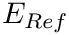and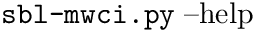be two conformations of a molecule of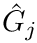atoms. We note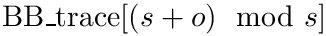the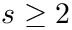coordinates of the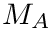-th atom of the conformation. The rigid registration ofoveris denoted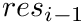, and its coordinates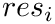. The least-RMSD ofandis the root mean square distance between coordinates ofand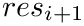: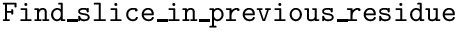The rigid registration is performed using the package Point_cloud_rigid_registration_3. Note that this rigid registration takes only account for rotations and translations. In this package, we also need to take account for chirality, so that we need in addition a mirror transformation in the registration.

The least-RMSD is defined over conformations with cartesian coordinates.

subsection sec-mol-dist-implementation-angle Angular distance
While the l-RMSD is a distance between two conformations in a Euclidean space, the angular distance is defined in a space of angles. Saying that, the coordinates of particles are internal coordinates and vary in the interval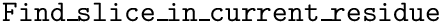. The angular distance is a RMSD where the pairwise distances between particles is defined over.
The angular distance is defined over the angles of conformations with internal coordinates.

# Implementation: other distances

## RMSD internal distance

The previous distances were RMSD defined for pairs of particles. Another way to compare conformation is to compute the RMSD between all possible pairs of particles of one conformation with all matching pairs of particles of the other conformation. The resulting distance is called the RMSD internal distance.

The RMSD internal distance is defined over conformations with cartesian coordinates.

## Other distances

This package offers also the possibility to plug any executable that takes two input D dimensional points and returns a single value distance. In this way, any other kind of distance, that is externally defined, can be used in the SBL.

The coordinate system to use with the conformations depends on the definition of the distance in the external program.

# Design

## Alignment

Except for the distance described in section Other distances, all described molecular distances require an alignment process to match particles of the two input conformations.

The concept MolecularAlignment is a simple functor taking as input the position of a particle in a conformation and a boolean tag indicating the direction of the alignment (first conformation to second, or opposite direction). It then returns the position of the input particle in the other conformation. The default functor is SBL::CSB::Molecular_alignment_default and simply returns the input position, assuming that both conformations are already aligned.

## Particles

The l-RMSD and the RMSD internal distances require both a geometric definition of a particle. As mentionned, a particle is represented by a 3D point. Thus, the concept GetParticle defines a functor that takes as input a conformation and a position, and returning a 3D point corresponding to the target particle. The default functor is SBL::CSB::T_Get_particle_default and simply returns a CGAL Point_3 structure of the ith particle in the input conformation.

## Least-RMSD

The l-RMSD is defined in the class SBL::CSB::T_Least_RMSD_cartesian< Conformation , GetParticle , MolecularAlignment > . The parameter Conformation is the representation of the input conformations and should be compliant with the CGAL::Point_d class of the CGAL library. The parameters GetParticle and MolecularAlignment were both previously described.

In addition, the class SBL::CSB::T_Least_RMSD_cartesian_with_chirality< Conformation , GetParticle , MolecularAlignment > computes the distances between mirror images of the input conformations, and returns the smallest distance.

subsubsection sec-mol-dist-implementation-design-angle Angular distance
The angular distance is defined in the class SBL::CSB::T_Squared_angular_internal_distance< Conformation , FT , MolecularAlignment > . The parameter FT is the number type used for computing the distance (by default, the double type), while the two other parameters were both previously described. Note that this functor returns the squared distance, avoiding the use of the square root operation, that might not be necessary.

## RMSD internal distance

The RMSD internal distance is defined in the class SBL::CSB::T_Squared_RMSD_internal_distance< Conformation , InternalDistance , GetParticle , MolecularAlignment > . The parameter InternalDistance is a functor for computing the distance between two particles in the same conformation. The three other parameters were all previously described. Note that this functor returns the squared distance, avoiding the use of the square root operation, that might not be necessary.

## Other distances

Externally defined distances can be wrapped using the class SBL::CSB::T_External_distance< Conformation , FT > . Parameters were all already described. The only additional requirements are :

• the name of the external executable computing the distance is named sbl-conf-distance.exe (this can be achieved with a symbolic link);
• the executable takes as input two text files, each representing a D dimensional point
• the executable prints in the standard output the distance as a single value.

# Examples

## Least-RMSD

The following example loads two conformations from two input files, and computes the least-RMSD between those two conformations.

#include <iostream>
#include <fstream>
#include <SBL/CSB/Least_RMSD_cartesian.hpp>
#include <CGAL/Cartesian_d.h>
#include <CGAL/Cartesian.h>
#include <SBL/Models/Conformation_traits_point_d.hpp>
#include <SBL/Models/Conformation_traits_vector.hpp>
//Works with primitive types
typedef CGAL::Cartesian_d<double> K;
//typedef K::Point_d Conformation;
typedef std::vector<K::FT> Conformation;
int main(int argc, char *argv[])
{
//Read 3D points from an input file
if(argc < 3) return -1;
Conformation p, q;
std::ifstream in_p(argv);
in_p >> p;
in_p.close();
std::ifstream in_q(argv);
in_q >> q;
in_q.close();
LRMSD distance;
std::cout << "LRMSD: " << distance(p, q) << std::endl;
return 0;
}
Model of Distances for defining distance between two conformations.
Definition: Least_RMSD_cartesian.hpp:84
p
Definition: extract-wales-graph.py:74
subsection sec-mol-dist-examples-angle Angular distance
The following example loads two conformations from two input files, and computes the angular distance between those two conformations.
#include <iostream>
#include <fstream>
#include <SBL/CSB/Squared_angular_internal_distance.hpp>
#include <CGAL/Cartesian_d.h>
#include <CGAL/Cartesian.h>
#include <SBL/Models/Conformation_traits_point_d.hpp>
//Works with primitive types
typedef CGAL::Cartesian_d<double> K;
typedef K::Point_d Conformation;
int main(int argc, char *argv[])
{
//Read 3D points from an input file
if(argc < 3) return -1;
Conformation p, q;
std::ifstream in_p(argv);
in_p >> p;
in_p.close();
std::ifstream in_q(argv);
in_q >> q;
in_q.close();
Distance distance;
std::cout << "Angular distance : " << CGAL::sqrt(distance(p, q)) << std::endl;
return 0;
}
Model of distance between angles of two conformations given with their internal coordinates.
Definition: Squared_angular_internal_distance.hpp:96

## RMSD internal distance

The following example loads two conformations from two input files, and computes the RMSD internal distance between those two conformations.

#include <iostream>
#include <fstream>
#include <SBL/CSB/Squared_RMSD_internal_distance.hpp>
#include <CGAL/Cartesian_d.h>
#include <CGAL/Cartesian.h>
#include <SBL/Models/Conformation_traits_point_d.hpp>
//Works with primitive types
typedef CGAL::Cartesian_d<double> K;
typedef K::Point_d Conformation;
typedef CGAL::Cartesian<double>::Compute_squared_distance_3 Internal_distance_base;
struct Internal_distance : public Internal_distance_base
{
typedef double FT;
typedef CGAL::Cartesian<double>::Point_3 Point;
};
int main(int argc, char *argv[])
{
Conformation p, q;
std::ifstream in_p(argv);
in_p >> p;
in_p.close();
std::ifstream in_q(argv);
in_q >> q;
in_q.close();
RMSD distance;
std::cout << "RMSD for internal distances: " << CGAL::sqrt(distance(p, q)) << std::endl;
return 0;
}
Model of Distance for defining distance between two conformations.
Definition: Squared_RMSD_internal_distance.hpp:87
RMSD distance between two 3D point clouds.
Definition: RMSD_cartesian_3.hpp:107

## Other distances

The following example loads two conformations from two input files, and computes the distance from an external executable called sbl-conf-distance.exe.

#include <iostream>
#include <fstream>
#include <SBL/CSB/External_distance.hpp>
#include <CGAL/Cartesian_d.h>
#include <SBL/Models/Conformation_traits_point_d.hpp>
typedef CGAL::Cartesian_d<double> K;
typedef K::Point_d Conformation;
int main(int argc, char *argv[])
{
Conformation p, q;
std::ifstream in_p(argv);
in_p >> p;
in_p.close();
std::ifstream in_q(argv);
in_q >> q;
in_q.close();
Distance distance;
std::cout << "External distance : " << distance(p, q) << std::endl;
return 0;
}
Distance computed from an external executable (sbl-conf-distance.exe) Distance computed from an exter...
Definition: External_distance.hpp:55

# Applications

This package also offers a variety of programs performing different tasks related to the least-RMSD:

• sbl-lrmsd-for-pdb-pair.exe : computes the least-RMSD between two conformations of a molecule defined in two PDB files passed as arguments. The chains loaded can be selected.
• sbl-lrmsd-for-pdb-list.exe : computes the least-RMSD between any two conformations of a molecule defined in PDB files listed in a given text file.
• sbl-lrmsd-for-pdb-models.exe : computes the least-RMSD between any two models found in a given PDB file.
• sbl-binet-cauchy-for-pdb-pairs.exe : computes the Binet Cauchy kernel score between two conformations of a molecule defined in two PDB files passed as arguments. The chains loaded can be selected.
• sbl-binet-cauchy-for-pdb-list.exe : computes the Binet Cauchy kernel score between any two conformations of a molecule defined in PDB files listed in a given text file.
• sbl-lrmsd-all-against-one.exe : computes the least-RMSD between a conformation defined in a first file and a collection of conformations defined in a second file;
• sbl-lrmsd-all-pairs.exe : computes the matrix of least-RMSD between all possible distinct pairs of conformations defined in an input file;
• sbl-lrmsd-conformational-ensembles-intersection.exe : computes the common set of conformations between two input sets using the least-RMSD (two conformations are said identical if their distance is below an input treshold); the output is a file listing pairs of indices, each index representing the position of a matched conformation in the file it was defined;
• sbl-lrmsd-conformational-ensembles-centroid-per-atom.exe : computes a conformation where each particle is a centroid of all matching particles of a set of input conformations.
• sbl-lrmsd-subdomain-comparator.exe : Given a list of proteins (as PDB files) and a sub-domain label classification (as defined in MolecularSystemLabelsTraits) for each, computes the least-RMSD between all sub-domains which have equal labels accross partners.

# Jupyter demo

See the following jupyter notebook:

• Jupyter notebook file
• Molecular_distances

# Molecular distances¶

The following sections provide examples for the following executables:

• sbl-lrmsd-for-pdb-pair.exe

## sbl-lrmsd-for-pdb-pair.exe: comparing structures from two PDB files¶

In the following, we compare two structure of the SARS-COV-2 receptor binding domain RBD.

In the options, note in particular:

• -- load-chains : to specify the chains of interest
• -- grain-mode: 0:Calpha 1:backbone atoms: 2: all heavy atoms
• -xyz: to check the coordinates of the atoms involved in the lRMSD calculation. Usefull for a sanity check in the presence of gaps in the chains and/or alternate locations, etc.

In the output, the following is noticeable:

• The program returns the lRMSD and the number of atoms involved (which depends on the grain mode)
• The presence of atoms with alternate locations
• The selection of the commons atoms to both chains (same resid, same atom name)
In :
# A Small function launching the calculation for a pair of PDB files
from subprocess import Popen, PIPE
from subprocess import check_output, CalledProcessError, STDOUT
import subprocess

def cmp_structures(exe, pdb1, chains1, pdb2, chains2, grain_mode):

cmd = [exe, "-f", pdb1, "--load-chains", chains1, "-f", pdb2, "--load-chains", chains2, "--grain-mode", grain_mode, "-v", "--xyz"]
s=subprocess.check_output(cmd, encoding='UTF-8')
print(s)


### Comparison based on Calpha atoms only: grain mode equal to 0¶

In :
 cmp_structures("sbl-lrmsd-for-pdb-pair.exe", "data/6w41.pdb", "C", "data/6m0j.pdb", "E", "0")

(ESBTL-DEBUG) Lines read 1623
Number of loaded PDB files: 2
Details for each file:
-- file 1 data/6w41.pdb:
-- -- Number of models: 1
-- -- Number of loaded atoms: 1543
-- -- Number of loaded SS bonds: 9
-- -- Number of discarded waters: 0
-- -- Number of discarded hetatoms: 30
-- -- Number of discarded hydrogens: 0
-- -- Number of discarded atoms: 3363
-- -- No alternate location
-- -- Number of atoms with not null occupancy < 1: 0
-- -- Number of atoms with null occupancy: 0
-- file 2 data/6m0j.pdb:
-- -- Number of models: 1
-- -- Number of loaded atoms: 1550
-- -- Number of loaded SS bonds: 7
-- -- Number of discarded waters: 66
-- -- Number of discarded hetatoms: 57
-- -- Number of discarded hydrogens: 0
-- -- Number of discarded atoms: 4883
-- -- 2 alternate locations: A B
-- -- Selected alternate location: A
-- -- Number of atoms with not null occupancy < 1: 0
-- -- Number of atoms with null occupancy: 0
lRMSD:0.711056 #atoms:194



### Comparison based on all backbone atoms: grain mode equal to 1¶

In :
 cmp_structures("sbl-lrmsd-for-pdb-pair.exe", "data/6w41.pdb", "C", "data/6m0j.pdb", "E", "1")

(ESBTL-DEBUG) Lines read 1623
Number of loaded PDB files: 2
Details for each file:
-- file 1 data/6w41.pdb:
-- -- Number of models: 1
-- -- Number of loaded atoms: 1543
-- -- Number of loaded SS bonds: 9
-- -- Number of discarded waters: 0
-- -- Number of discarded hetatoms: 30
-- -- Number of discarded hydrogens: 0
-- -- Number of discarded atoms: 3363
-- -- No alternate location
-- -- Number of atoms with not null occupancy < 1: 0
-- -- Number of atoms with null occupancy: 0
-- file 2 data/6m0j.pdb:
-- -- Number of models: 1
-- -- Number of loaded atoms: 1550
-- -- Number of loaded SS bonds: 7
-- -- Number of discarded waters: 66
-- -- Number of discarded hetatoms: 57
-- -- Number of discarded hydrogens: 0
-- -- Number of discarded atoms: 4883
-- -- 2 alternate locations: A B
-- -- Selected alternate location: A
-- -- Number of atoms with not null occupancy < 1: 0
-- -- Number of atoms with null occupancy: 0
lRMSD:0.684967 #atoms:582



### Comparison based on all heavy atoms: grain mode equal to 2¶

In :
 cmp_structures("sbl-lrmsd-for-pdb-pair.exe", "data/6w41.pdb", "C", "data/6m0j.pdb", "E", "2")

(ESBTL-DEBUG) Lines read 1623
Number of loaded PDB files: 2
Details for each file:
-- file 1 data/6w41.pdb:
-- -- Number of models: 1
-- -- Number of loaded atoms: 1543
-- -- Number of loaded SS bonds: 9
-- -- Number of discarded waters: 0
-- -- Number of discarded hetatoms: 30
-- -- Number of discarded hydrogens: 0
-- -- Number of discarded atoms: 3363
-- -- No alternate location
-- -- Number of atoms with not null occupancy < 1: 0
-- -- Number of atoms with null occupancy: 0
-- file 2 data/6m0j.pdb:
-- -- Number of models: 1
-- -- Number of loaded atoms: 1550
-- -- Number of loaded SS bonds: 7
-- -- Number of discarded waters: 66
-- -- Number of discarded hetatoms: 57
-- -- Number of discarded hydrogens: 0
-- -- Number of discarded atoms: 4883
-- -- 2 alternate locations: A B
-- -- Selected alternate location: A
-- -- Number of atoms with not null occupancy < 1: 0
-- -- Number of atoms with null occupancy: 0
lRMSD:1.30741 #atoms:1536